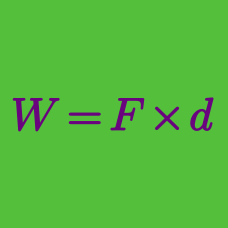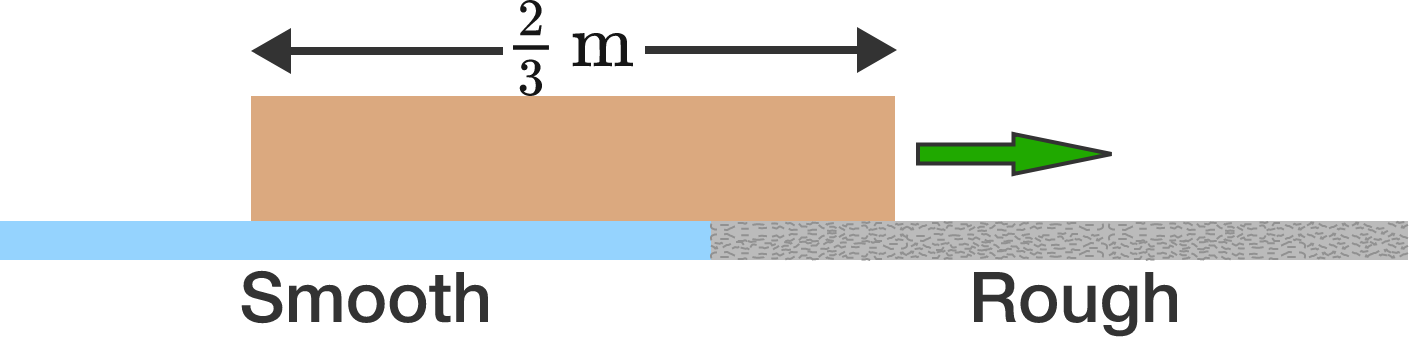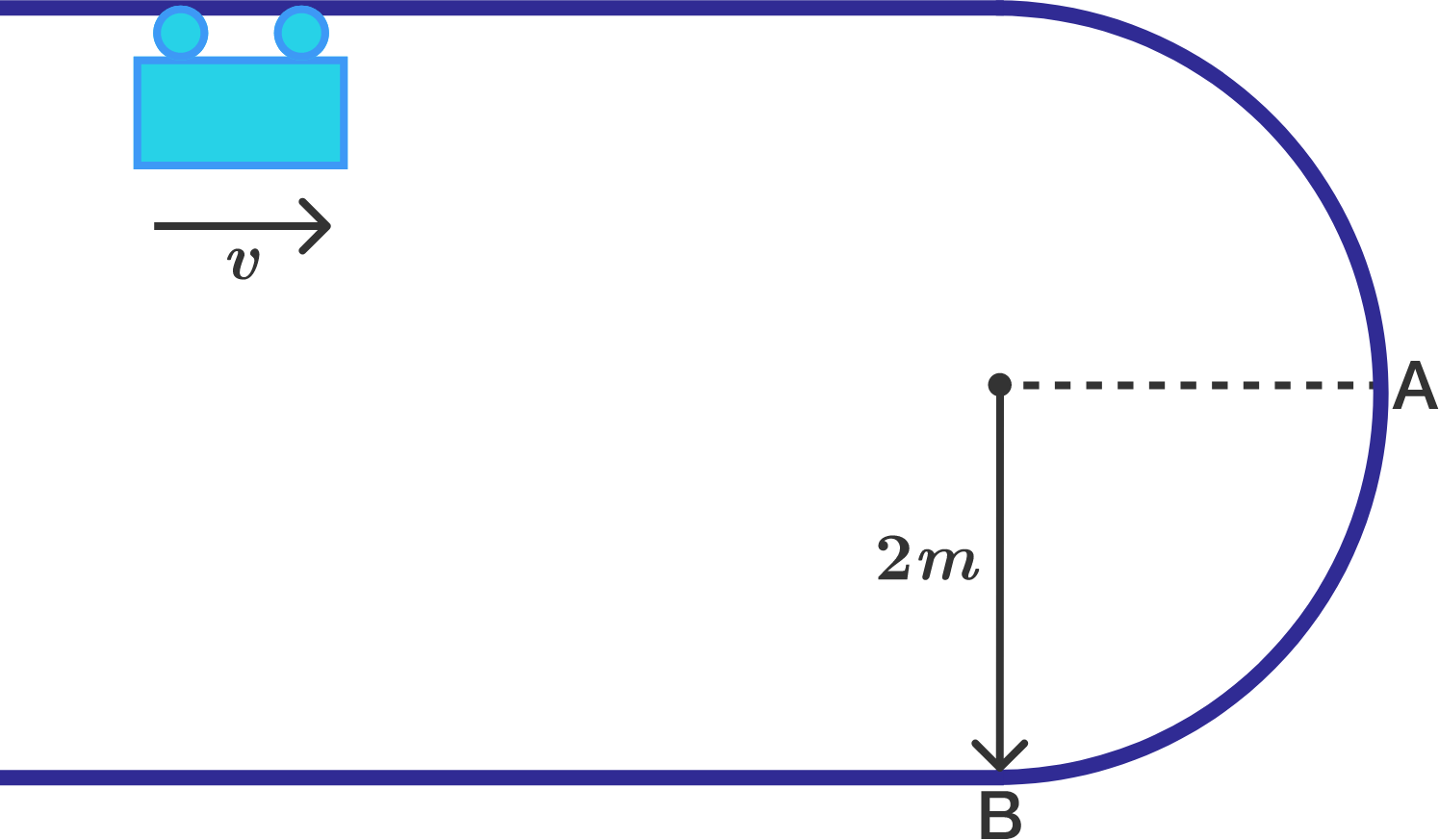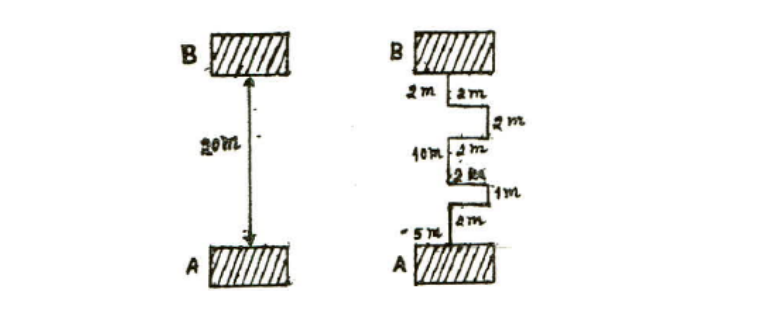Classical Mechanics

# Work: Level 3 ChallengesA rectangular box of length $\frac{2}{3} \text{m}$ is initially traveling at $2 \text{ m/s},$ with its entire length over the smooth (perfectly frictionless) blue surface (shown above). The box gradually moves onto a rough surface (gray) and stops the instant that its entire length is positioned within the rough region.

Determine the coefficient of kinetic friction.


Details and Assumptions:

• $g = 10\text{ m/s}^2.$
• The pressure at the bottom of the box is always uniform over its area.

A $1.2\text{ kg}$ mass is projected down a rough semi-circular vertical track of radius $2.0 \text{ m}$, as shown in the diagram below. The speed of the mass at point $A$ is $3.2\text{ m/s}$, and that at point $B$ is $6.0 \text{ m/s}$.

How much work is done on the mass between $A$ and $B$ by the force of friction?A $1\text{ kg}$ object is lifted from point A to point B through two different pathways as shown in the figure above. What are the respective amounts of work done in the left and right figures?

The gravitational acceleration is $g=9.8\text{ m/s}^2.$

Two forces $\left(6\hat{i} + 2\hat{j} - 3\hat{k}\right) \si{\newton}$ and $\left(5\hat{i} - 3\hat{j} + 7\hat{k}\right) \si{\newton}$ act on an object that moves on a frictionless surface and, in doing so, displace it from $\left(2\hat{i} + 3\hat{j} - 5\hat{k}\right) \si{\metre}$ to $\left(-3\hat{i} - 3\hat{j} + 4\hat{k}\right) \si{\metre}$.

Find the magnitude of the work done on the object, $W$ in joules.

A locomotive of mass $m$ starts moving so that its velocity varies according to the law $v=k \sqrt{s}$, where $k$ is a constant and $s$ is the distance covered. Find the total work performed by all the forces which are acting on the locomotive during the first $t$ seconds, after the beginning of motion.

• If $W=\large\frac { m{ k }^{ \alpha }{ t }^{ \beta } }{ \mu }$, find $\alpha +\beta +\mu$.
×